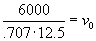Projectile Motion

From physics and trigonometry we can derive the following parametric equations for the projectile. We will assume the projectile is fired at an angle of 45° from the horizontal. (This angle maximizes the range.) Let v0 = initial velocity.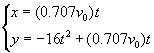To hit a target located at the point (6000, 3500), we need to set x = 6000 and y = 3500 and solve the resulting system of equations.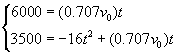Solve the x equation for v0: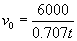Substituting into the y equation: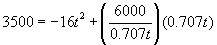Simplify: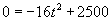Using the x equation